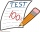Camp

In the camp are children. 1/2 went on a trip, 1/4 went to bathe and 38 children remained in the room. How many children are in camp?

Result

x =  152

Solution:Leave us a comment of this math problem and its solution (i.e. if it is still somewhat unclear...):Be the first to comment!To solve this verbal math problem are needed these knowledge from mathematics:

Need help calculate sum, simplify or multiply fractions? Try our fraction calculator. Do you have a linear equation or system of equations and looking for its solution? Or do you have quadratic equation?

Next similar math problems:

1. Examination 2In an examination, a teacher awards 2 marks for every correct answer and subtract a mark for every wrong answer. If there are 20 questions and a child scored 25 marks how many correct answer did the child get?
2. Lengths of the poolMiguel swam 6 lengths of the pool. Mat swam 3 times as far as Miguel. Lionel swam 1/3 as far as Miguel. How many lengths did mat swim?
3. Passenger boatTwo-fifths of the passengers in the passenger boat were boys. 1/3 of them were girls and the rest were adult. If there were 60 passengers in the boat, how many more boys than adult were there?
4. Pizza 4Marcus ate half pizza on monday night. He than ate one third of the remaining pizza on Tuesday. Which of the following expressions show how much pizza marcus ate in total?
5. The quotientThe quotient of g and 55 is the same as 279. What is g?
6. Fractions 3Calculate 1/9 of 27:
7. Pizza 5You have 2/4 of a pizza and you want to share it equally between 2 people how much pizza does each person get?
8. What isWhat is the value of the smaller of a pair of numbers for which their sum is 78 and their division quotients are 0.3?
9. Equation 15Solve equation with variables on both sides:
10. EquationFind x: x + 1/2 = 1/3
11. Unknown numberI think the number. I'll reduce it to its one-third. The result is then increased by one-third, and I get the number 12.
12. Eq-fracSolve the following equation with fractions: h + 1/3 =5/3
13. Missing numberBlank +1/6 =3/2 find the missing number
14. Negative in equation2x + 3 + 7x = – 24, what is the value of x?
15. Write decimalsWrite in the decimal system the short and advanced form of these numbers: a) four thousand seventy-nine b) five hundred and one thousand six hundred and ten c) nine million twenty-six
16. Simple equation 8Solve the following equation: 36=-(1+7x)-6(-7-x)
17. Fraction to decimalWrite the fraction 3/22 as a decimal.